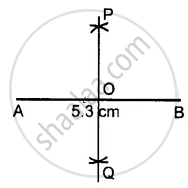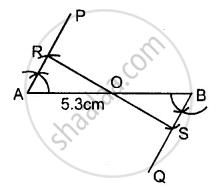# Draw a Line Segment Ab of Length 5.3 Cm. Using Two Different Methods Bisect Ab. - Mathematics

Sum

Draw a line segment AB of length 5.3 cm. Using two different methods bisect AB.

#### Solution

Steps of Construction :1. Draw a line segment AB = 5.3 cm
2. With A as centre and radius equal to more than half of AB, draw arcs on both sides of AB.
3. With B as the centre and with the same radius as taken in step 2, draw arcs on both sides of AB.
4. Let the arcs intersect each other at points P and Q.
5. Join P and Q.
6. The line PQ cuts the given line segment AB at point O.
Thus, PQ is a bisector of AB such that
OA = OB = 1/2AB

Second MethodSteps of Construction :

1. Draw the given line segment AB = 5.3 cm.
2. At A, construct ∠PAB of any suitable measure. Then ∠PAB = 60° construct ∠QBA = 60°
3. 3. From AP, cut AR of any suitable length and from BQ; cut BS = AR.
4. Join R and S
5. Let RS cut the given line segment AB at the point O.
Thus RS is a bisector of AB such that OA = OB = 1/2AB
Concept: Construction of an Angle - To Draw the Bisector of a Given Angle
Is there an error in this question or solution?
Chapter 18: Constructions - Exercise 18 (B) [Page 204]

#### APPEARS IN

Selina Concise Mathematics Class 8 ICSE
Chapter 18 Constructions
Exercise 18 (B) | Q 1 | Page 204
Share